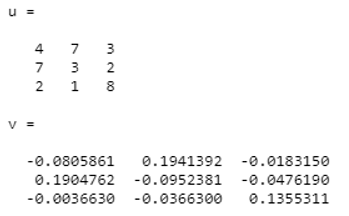Categories

# Matlab Inverse Function

In Matlab, the Inverse function is obtained by applying the ‘ finverse ’ command. This command returns the value of the given function variable. In finverse f stands for function and after finverse, there will be parameters or argument list inside the brackets depending upon the requirement. if function depends only on one variable then inside bracket there will be one argument which if function (f) and if function depends on two arguments then there will be two parameters inside the brackets.

## Syntax:

There are two syntaxes, it varies depending upon variable dependency.

``Y = finverse (f)``

This command is used if there are multiple variables in the function.

``Y = finverse (f, var)``

this command is used if there is only one dependent variable in function.

## Examples of Matlab Inverse Function

The examples of Matlab Inverse functions are given below:

## 1. The inverse of cos x

``````sym x ;
Y ( x )  =
Cos ( x )
z = finverse ( y )
Z ( x ) =
a cos ( x  )``````

## 2. Inverse of 1/tan(x)

``````sym x ;
y ( x ) = 1 / tan ( x )
Y ( x ) =
1 / tan ( x )
z = finverse ( y )
Z ( x ) =
a tan ( 1 / x )</code``````

## 3. The inverse of exponential ( x – 5 y )

``````syms  x  y
finverse ( exp ( x - 5 * y) , x )
ans  =
5 * y + log ( x )``````

## 4. The inverse of log (x-y)

``````syms  x  y
finverse ( log ( x – y ), y )
ans =
x – exp ( y )``````

## The Inverse of Matrix

To find the inverse of any matrix ‘inv’ command is used. Consider two variables u and v independently. Where v is output var and u is input variable. Then command to find inverse will be v = inv ( u ). Here u^-1 is almost equal to inv(u).

### 1. Matlab code to find the inverse of the above matrix;

Consider matrix u ;

u=[ 4 7 3; 7 3 2;2 1 8]

v=inv(u)To obtain the inverse of the matrix there is one condition, the input matrix must be ‘square matrix’. otherwise, it will give the error. let see one example of the odd matrix (rectangular matrix).

## 2. Matlab code to obtain the inverse

Consider matrix U,

u=[ 6 4 2 9; 6 4 3 1;8 6 4 2]

v=inv(u)

### 3. Matlab code to obtain inverse ;

Now consider,

u=[43 56 98 65 43;54 63 2 43 5;8 4 32 42 12;54 6 78 54 3;6 5 43 38 32]

v=inv(u)